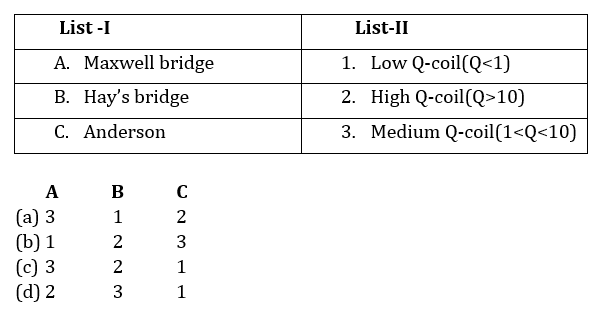Engineering Jobs   »   DFCCIL ELECTRICAL QUIZ,

# DFCCIL’21 EE: Daily Practices Quiz 19-July-2021

Each question carries 1 mark.
Negative marking: 1/4 mark
Total Questions: 06
Time: 08 min.

Q1. The short circuit test in a transformer is used to determine……
(a) Eddy current
(b) Hysteresis loss
(c) Copper loss at any load
(d) Iron loss at any load

Q2. Two coils with self-inductances 1 H and 2 H having a mutual inductance of 1 H between them carry currents of 2 A and √2 A respectively. The energy stored in the field, in joules, is
(a) 2(1+√2)
(b) 3(1+√2)
(c) 2(2+√2)
(c) 3(2+√2)

Q3. Ferranti effect happens in a transmission line when line is
(d) none of the above

Q4. What is the ratio of starting torque and maximum torque of a 3-phase, 50 Hz, 4 pole induction motor for maximum torque at 1200 rpm?
(a) 0.5
(b) 0.6
(c) 0.421
(d) 0.384

Q5. If the gain of the open-loop system is doubled, the gain margin
(a) Becomes half
(b) Gets doubled
(c) Becomes one-fourth
(d) Is not affected

Q6. Match the followingSOLUTIONS
S1. Ans.(c)
Sol. SC test of transformer:
Used to determine copper loss or ohmic loss
LV side short circuited
Instruments kept on HV side
Also determines equivalent resistance and leakage reactance

S2. Ans.(c)
Sol. ACQ: L_1=1 H,L_2=2 H & M=1 H
& i_1=2 A,i_2=√2 A
Energy stored=[1/2 L_1 i_1^2+1/2 L_2 i_2^2+Mi_1 i_2 ]J
∴E=[1/2×1×4+1/2×2×2+1×2×√2]=4+2√2=2(2+√2) joules

S3. Ans.(b)
Sol.
Ferranti effect occurs for medium and long transmission line.
Due to Ferranti effect, receiving end voltage is more than sending end voltage.ie.V_R>V_S

S4. Ans.(d)
Sol. N_s=120f/P=(120×50)/4=1500 rpm
∴s=(N_s-N_r)/N_s =(1500-1200)/1500=0.2
∴T_st/T_m =2/(s+1/s)=2/(0.2+1/0.2)=2/5.2=0.384

S5. Ans.(a)
Sol. Gain margin is calculated at phase cross over frequency. At phase cross-over frequency phase of G(jω) is -180⁰.
GM=1/X
If X becomes doubled then the GM becomes half.

S6. Ans.(c)
Sol. Bridges used for measurement of inductance:
Anderson bridge (Q<1).
Maxwell bridge (1<Q<10).
Hay bridge (Q>10).

Sharing is caring!

Thank You, Your details have been submitted we will get back to you.